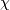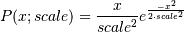# numpy.random.rayleigh¶

numpy.random.rayleigh(scale=1.0, size=None)

Draw samples from a Rayleigh distribution.

Theand Weibull distributions are generalizations of the Rayleigh.

Parameters: scale : scalar Scale, also equals the mode. Should be >= 0. size : int or tuple of ints, optional Output shape. If the given shape is, e.g., (m, n, k), then m * n * k samples are drawn. Default is None, in which case a single value is returned.

Notes

The probability density function for the Rayleigh distribution isThe Rayleigh distribution would arise, for example, if the East and North components of the wind velocity had identical zero-mean Gaussian distributions. Then the wind speed would have a Rayleigh distribution.

References

 [R259] Brighton Webs Ltd., “Rayleigh Distribution,” http://www.brighton-webs.co.uk/distributions/rayleigh.asp
 [R260] Wikipedia, “Rayleigh distribution” http://en.wikipedia.org/wiki/Rayleigh_distribution

Examples

Draw values from the distribution and plot the histogram

>>> values = hist(np.random.rayleigh(3, 100000), bins=200, normed=True)


Wave heights tend to follow a Rayleigh distribution. If the mean wave height is 1 meter, what fraction of waves are likely to be larger than 3 meters?

>>> meanvalue = 1
>>> modevalue = np.sqrt(2 / np.pi) * meanvalue
>>> s = np.random.rayleigh(modevalue, 1000000)


The percentage of waves larger than 3 meters is:

>>> 100.*sum(s>3)/1000000.
0.087300000000000003


#### Previous topic

numpy.random.power

#### Next topic

numpy.random.standard_cauchy